Courses

# Long Answers - Number System Class 9 Notes | EduRev

## Class 9 : Long Answers - Number System Class 9 Notes | EduRev

The document Long Answers - Number System Class 9 Notes | EduRev is a part of the Class 9 Course Mathematics (Maths) Class 9.
All you need of Class 9 at this link: Class 9

Q.1. Find six rational numbers between 1 and 2.
Solution.
Let x = 1 and y = 2
⇒   y > x
Here n = 6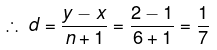∴ The six rational numbers between 1 and 2 are: (x + d), (x + 2d), (x + 3d), (x + 4d), (x + 5d) and (x + 6d).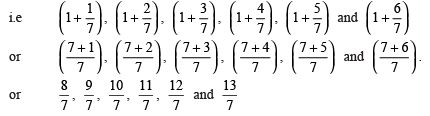Q.2. Find five rational numbers between 0.6 and 0.8.
Solution.
Let x = 0.6 and y = 0.8
⇒   y > x
Here, n = 5
∴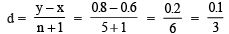Now, the five rational numbers between 0.6 and 0.8 are: (x + d), (x + 2d), (x + 3d), (x + 4d), and (x + 5d).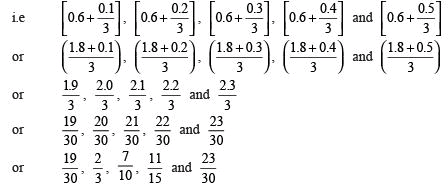Thus, the required five rational numbers between 0.6 and 0.8 are: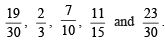Q.3. If ‘a’ and ‘b’ are rational numbers and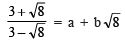then find the value of ‘a’ and ‘b’.
Solution.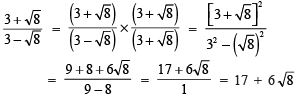Now⇒ a + b√8 = 17 +6√8
⇒ a = 17 and b = 6

Offer running on EduRev: Apply code STAYHOME200 to get INR 200 off on our premium plan EduRev Infinity!

## Mathematics (Maths) Class 9

46 videos|323 docs|108 tests

,

,

,

,

,

,

,

,

,

,

,

,

,

,

,

,

,

,

,

,

,

;